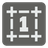[old] The Hamming Distance
Undefined
English FR HU RU UK

The Hamming distance between two binary integers is the number of bit positions that differs (read more about the Hamming distance on Wikipedia). For example:

```    117 = 0 1 1 1 0 1 0 1
17 = 0 0 0 1 0 0 0 1
H = 0+1+1+0+0+1+0+0 = 3

```

You are given two positive numbers (N, M) in decimal form. You should calculate the Hamming distance between these two numbers in binary form.

Input: Two arguments as integers.

Output: The Hamming distance as an integer.

Example:

```hammingDistance(117, 17) == 3
hammingDistance(1, 2) == 2
hammingDistance(16, 15) == 5
```

How it is used: This is a basis for Hamming code and other linear error-correcting programs. The Hamming distance is used in systematics as a measure of genetic distance. On a grid (ie: a chessboard,) the Hamming distance is the minimum number of moves it would take a rook to move from one cell to the other.

Precondition:
0 < n < 106
0 < m < 106